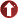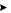Mathematics and Statistics
Master of Science in Mathematics and StatisticsFields of Specialization Mathematics Statistics Dean Ian D. Brindle Faculty of Mathematics & Science Associate Dean Greg Finn Faculty of Mathematics & Science Graduate Faculty Professor Emeritus Howard Bell (Mathematics) Professors Hichem Ben-El-Mechaiekh (Mathematics), Mei Ling Huang (Mathematics), Ronald A. Kerman (Mathematics), Jan Vrbik (Mathematics), Thomas Wolf (Mathematics) Associate Professors Stephen Anco (Mathematics), Henryk Fuk (Mathematics), Omar Kihel (Mathematics), Yuanlin Li (Mathematics) Assistant Professors Chantal Buteau (Mathematics), Xiaojian Xu (Mathematics), Wai Kong (John) Yuen (Mathematics) Adjunct Professors Vladimir Sokolov (Landau Institute) Graduate Program Director Stephen Anco sanco@brocku.ca General Inquiries E-mail: mathstatgrad@brocku.ca Administrative Assistants Margaret Thomson, Josephine McDonnell Mackenzie Chown J415 905-688-5550, extension 3300 http://www.brocku.ca/mathematics/mscprog/index.php
Program DescriptionThe MSc program aims to provide students with an intensive advanced education in areas of Mathematics and Statistics in preparation for further graduate studies or the job market. Students will choose a concentration in either Mathematics or Statistics. The Mathematics concentration provides students with advanced training in areas of active research and current applicability in algebra and number theory, computer algebra algorithms, dynamical systems, partial differential equations, functional analysis, mathematical music theory, solitons and integrable systems, topology, and (as a bridge with the Statistics Concentration) probability theory and stochastic processes. The Statistics concentration provides students with solid training in advanced statistical analysis and in computational methods and applications to stochastic models. The program offers two options: a thesis option (intended normally for students planning to pursue further graduate studies) and a project option (intended normally for those planning to join the job market).
Fields of SpecializationParticipating faculty are engaged in active research in the following areas of specialization:
MathematicsComputational methods for solving algebraic and differential systemsCryptographyFourier and wavelets analysisFunctions spaces and wavelets applied to partial differential equationsGroup and ring theoryHigh performance parallel computingMathematical music theoryMathematical physics and General RelativityNonlinear functional analysis and applications to optimization, game theory, mathematical economics, and differential systemsProbability and measure theorySimulation techniques and modeling with discrete dynamical systemsSolitons and integrability of partial differential equationsSymmetry analysis and computer algebra applied to nonlinear differential equations

StatisticsStatistical Inference Methods and ApplicationsComputational methods and applications to stochastic modelsConvergence and Efficiency of Markov Chain Monte Carlo Algorithms

Admission RequirementsSuccessful completion of an Honours Bachelor's degree, or equivalent, in Mathematics, or Statistics, or a related field, with an overall average of not less than B+. Agreement from a faculty advisor to supervise the student is also required for admission to the program. The Graduate Admissions Committee will review all applications and recommend admission for a limited number of suitable candidates. Those lacking sufficient background preparation may be required to complete a qualifying term or year to upgrade their applications. Completion of a qualifying term or year does not guarantee acceptance into the program. Part-time study is available.
Degree RequirementsThe program requirements in Mathematics and Statistics include successful completion of core and specialization courses, and a thesis (MATH 5F90) or a project (MATH 5P99). Students in the thesis option are required to complete four half-credit courses: two core concentration courses, one specialization course, and a graduate seminar. They must also write a thesis that demonstrates a capacity for independent work of acceptable scientific calibre. Students in the project option are required to complete six half-credit courses. For students in Mathematics, four core concentration courses, one specialization course, and a graduate seminar; for students in Statistics, four core concentration courses, one specialization course, and an additional core or specialization course. In addition, each student must complete a project under the supervision of a faculty member. The project is more practical in nature and must demonstrate a capacity for synthesis and understanding of concepts and techniques related to a specific topic. Each student will consult with his or her Supervisor when planning a program of study and choosing courses and must receive approval from the Graduate Program Director. Core courses for the Mathematics concentration include:MATH 5P10 Modern AlgebraMATH 5P20 Computational Methods for Algebraic and Differential SystemsMATH 5P30 Dynamical SystemsMATH 5P40 Functional AnalysisMATH 5P50 Algebraic Number TheoryMATH 5P60 Partial Differential EquationsMATH 5P70 TopologyMATH 5P87 Probability and Measure TheoryMATH 5P94 MSc Mathematics Seminar
Specialization courses for the Mathematics concentration include:MATH 5P05 Introduction to WaveletsMATH 5P09 Solitons and Nonlinear Wave EquationsMATH 5P11 Group RingsMATH 5P21 Heuristic and Parallel Techniques for Algebraic and Differential SystemsMATH 5P31 Mathematical Models of Complex SystemsMATH 5P32 Mathematical Foundations of Statistical PhysicsMATH 5P41 Nonlinear Functional Analysis IMATH 5P42 Nonlinear Functional Analysis IIMATH 5P44 Wavelet Bases in Functions Spaces With ApplicationsMATH 5P61 Symmetry Analysis and Conservation Law MethodsMATH 5P63 Integrability and Formal Geometry of PDEsMATH 5P64 Geometric Topics in Mathematical PhysicsMATH 5P71 Advanced TopologyMATH 5P72 Mathematical Music TheoryMATH 5P84 Time Series Analysis and Stochastic ProcessesMATH 5P92 Cryptography and Number Theory
Core courses for the Statistics concentration include:MATH 5P81 Sampling TheoryMATH 5P82 Nonparametric StatisticsMATH 5P83 Mathematical Statistical InferenceMATH 5P84 Time Series Analysis and Stochastic ProcessesMATH 5P85 Mathematical Statistical InferenceMATH 5P86 Multivariate Statistics
Specialization courses for the Statistics concentration include:MATH 5P87 Probability and Measure TheoryMATH 5P88 Advanced StatisticsMATH 5P95 MSc Statistics Seminar
The normal duration of the M.Sc. program is twenty-four months. However, completion in twelve months is possible in the Statistics concentration.

FacilitiesEach graduate student will be provided with personal desk space and a desktop PC linked to the university network system. In addition, graduate students will have access to the Mathematics computer lab classroom equipped with 33 PCs as well as to computer labs located in the vicinity of the Mathematics Department. Software includes Maple 8 and 10, MatLab, Visual Studio, SAS, S-PLUS, SPSS and Minitab, computer language compilers (C, C++, Fortran), Tex/Latex. Students will have access to advanced computing facilities that includea number of dedicated local servers running special softwarea departmental 6-node clustera 42-node parallel (Beowulf) cluster of 3 GHz Pentium IV computers20 computer clusters (with more than 8000 CPUs in total) in the Ontario
High Performance Computing consortium SHARCNET, ranging from highly connected clusters to SMP (Shared Memory Processor) computers with large memory and 70TB of disk space.

Course Descriptions